• 傅里叶级数matlab编程
千次阅读
2021-05-08 06:30:06

傅里叶级数展开matlab 实现给个例子说明下：将函数

y=x*(x-pi)*(x-2*pi)，在(0,2*pi)的范围内傅里叶级数展开syms x fx=x*(x-pi)*(x-2*pi);

[an,bn,f]=fseries(fx,x,12,0,2*pi)%前12 项展开latex(f)%将f 转换成latex 代码an = [ 0, 0, 0, 0, 0, 0, 0, 0, 0, 0, 0, 0, 0] bn = [ -12, 3/2, -4/9, 3/16, -12/125, 1/18, -12/343, 3/128, -4/ 243, 3/250, -12/1331, 1/144] f =

12*sin(x)+3/2*sin(2*x)+4/9*sin(3*x)+3/16*sin(4*x)+12/ 125*sin(5*x)+1/18*sin(6

*x)+12/343*sin(7*x)+3/128*sin(8*x)+4/243*sin(9*x)+3/ 250*sin(10*x)+12/1331* sin(11*x)+1/144*sin(12*x) ans = 12\,\sin \left( x \right) +3/2\,\sin \left( 2\,x \right)

+4/9\,\sin \left( 3\,x \right) +3/16\,\sin \left( 4\,x \right) +{\frac {12}{125}}\,\sin \left( 5\,x \right) +1/18\,\sin

\left( 6\,x \right) +{\frac {12}{343}}\,\sin \left( 7\,x \right) +{\frac {3}{128}}\,\sin \left( 8\,x \right) +{\frac

{4}{243}}\,\sin \left( 9\,x \right) +{\frac {3}{250}}\,\sin \left( 10\,x \right) +{\frac {12}{1331}}\,\sin \left( 11\,x \right) +{\frac {1}{144}}\,\sin \left( 12\,x \right) function [an,bn,f]=fseries(fx,x,n,a,b) %傅里叶级数展开% %an 为fourier 余弦项系数%bn 为fourier 正弦项系数%f 为展开表达式%f 为给定函数%x 为自变量%n 为展开系

更多相关内容
•matlab
• 这里的程序绘制了用户给定 'Ck' 的周期信号图。 以下数据应由用户输入。 1. Ck 值（分别为 k=0、k= even 和 k=odd） 2. 周期函数的时间段3. 分辨率4. k的范围（实际上是-inf到+inf）我们只能给出合适的值让过程收敛...matlab
• 这个程序试图逼近函数 f(x) 从 -L 到 L 使用 quad（MATLAB 函数）的 m 项傅立叶级数matlab
• 河南工程学院理 学 院MATLAB(傅立叶级数的模拟)姓名：杨标班级：信息1241学号：201211002126实验目的： (1)用MATLAB模拟傅立叶级数 F(x)=a0/2+ 求a0,an,bn;(2)对矩形函数，三角函数求傅立叶级数；(作图)(3)绘制函数...

河南工程学院

理 学 院

MATLAB(傅立叶级数的模拟)

姓名：杨标班级：信息1241学号：201211002126

实验目的： (1)用MATLAB模拟傅立叶级数 F(x)=a0/2+ 求a0,an,bn;(2)对矩形函数，三角函数求傅立叶级数；(作图)(3)绘制函数及其傅立叶逼近实验分析(1)：将F(x)=a0/2+ 展开为傅立叶级数，既是求ai,bi 根据三角函数的正交性，可得：a0=;an=;bn=应用积分求解公式int源程序：clearsyms x nf=(“函数方程”) a0=int(f,x,-pi,pi)/pi an=int(f*cos(n*x),x,-pi,pi)/pibn=int(f*sin(n*x),x,-pi,pi)/pi例：求函数f(x)=x^3+5 clearsyms x nf=x^3+5a0=int(f,x,-pi,pi)/pian=int(f*cos(n*x),x,-pi,pi)/pibn=int(f*sin(n*x),x,-pi,pi)/pi运行后得：

实验分析(2)：其中函数为F(X)=X，求解图像及其傅里叶逼近。 展开为傅里??级数： F(x)=a0/2+ ，先求解其中的a0,an,bn源程序：function y=fly(f,k,l)%f为要展开的函数，k为要展开的项数,l为函数半周期syms x n;a0=int(f,x,-l,l)/lan=int(f*cos(n*pi*x/l),x,-l,l)/lbn=int(f*sin(n*pi*x/l),x,-l,l)/lfor n=1:k a(n)=int(f*cos(n*pi*x/l),x,-l,l)/l; b(n)=int(f*sin(n*pi*x/l),x,-l,l)/l;endg=0;for n=1:k s=a(n)*cos(n*pi*x/l)+b(n)*sin(n*pi*x/l); g=g+s; end y=a0/2+g

依次可以求得，1,3,5,7,10次的部分和，既可以做出图像。输入：x=-pi:0.01:pif1=2*sin(x)f3=2*sin(x)-sin(2*x)+2/3*sin(3*x)f5=2*sin(x)-sin(2*x)+2/3*sin(3*x)-1/2*sin(4*x)+2/5*sin(5*x)f7=2*sin(x)-sin(2*x)+2/3*sin(3*x)-1/2*sin(4*x)+2/5*sin(5*x)-1/3*sin(6*x)+2/7*sin(7*x)f10=2*sin(x)-sin(2*x)+2/3*sin(3*x)-1/2*sin(4*x)+2/5*sin(5*x)-1/3*sin(6*x)+2/7*sin(7*x)-1/4*sin(8*x)+2/9*sin(9*x)-1/5*sin(10*x) plot(x,x,x,f1,x,f3,x,f5,x,f7,x,f10)即可得图像：实验分析(3)：求以2π为周期的周期函数函数F(x)=(-1,x<0;1,x>0)的傅里叶系数展开，及逼近(作图)源程序：[A,B,y]=fseries(f,x,n,a,b)f为待展开函数，x为自变量；n为展开项数；[a,b]为展开区间；A,B为傅里叶系数；y为返回的展开式(a)function [A,B,F]=fseries(f,x,n,a,b)if nargin==3, a=-pi; b=pi; end %若输入为3个参数表示f(x)在[-pi,pi]内展开L=(b-a)/2;A=int(f,x,-L,L)/L; %f(x)在[-L,L]内积分B=[]; F=A/2;for i=1:n an=int(f*cos(i*pi*x/L),x,-L,L)/L; %积分求系数an bn=int(f*sin(i*pi*x/L),x,-L,L)/L; %积分求系数bn A=[A,an]; %记录所有an B=[B,bn]; %记录所有bn F=F+an*cos(i*pi*x/L)+bn*sin(i*pi*x/L); %得到n阶傅里叶展开式end(b)syms x;f=abs(x)/x; %给出待展开函数xx=[-p

展开全文• @[TOC]([Matlab-2]函数展开为傅里叶级数(Fourier Series)) 周期延拓 在遇到非周期函数尤其是一段函数时，我们常常将其周期延拓 在时域下，表达式为 如下方波的傅里叶级数为 数值法实现傅里叶级数展开（Realize ...

## 周期延拓

在遇到非周期函数尤其是一段函数时，我们常常将其周期延拓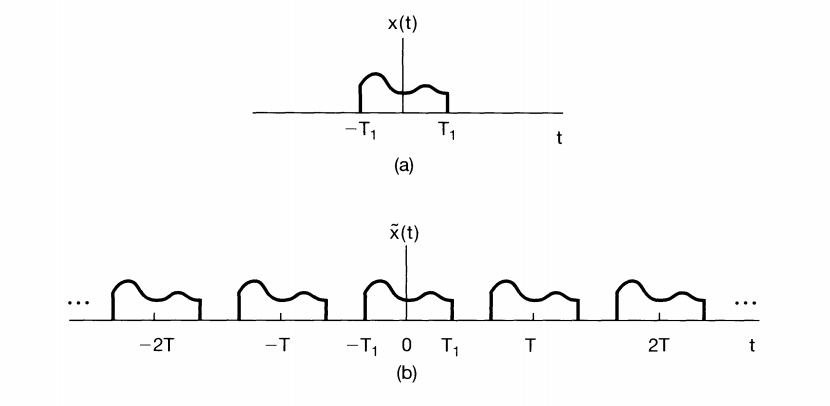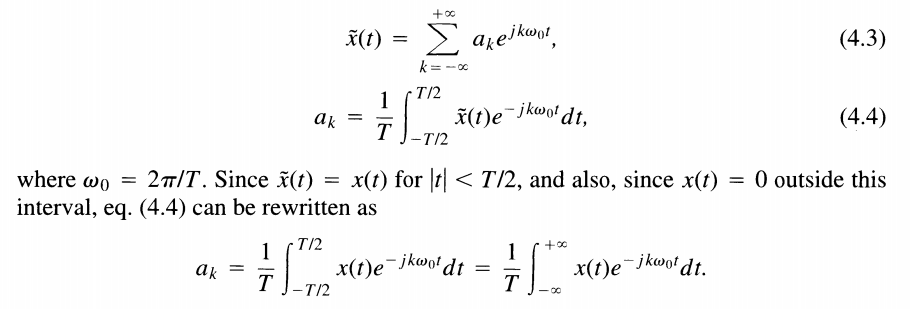在时域下，表达式为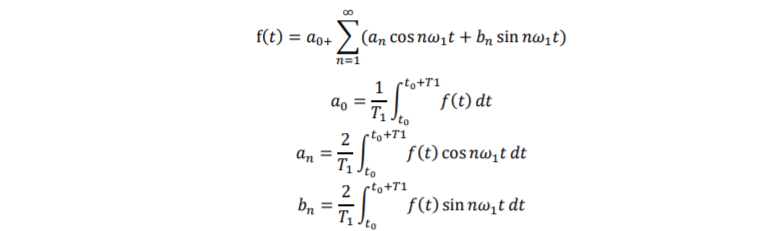如下方波的傅里叶级数为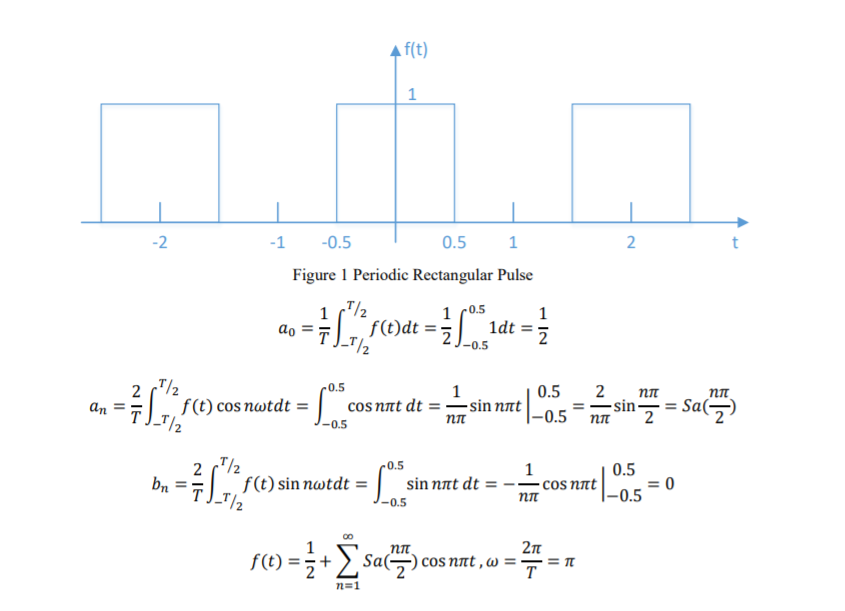## Loop形式

% numeric method: loop function, trapz
clear;clf;
T = 2; f = 1/T; w1 = 2*pi*f;
dt = 0.01;
t = -2:dt:2;
tao = -0.5:dt:0.5;
a0 = trapz(tao,tao.^0)/T;
f = a0;
N = input('N=');
an = zeros(1,N);
bn = zeros(1,N);
for n = 1:N
fcos = 1.*cos(n*w1*tao); an(n)=trapz(tao,fcos)*2/T;
fsin = 1.*sin(n*w1*tao); bn(n)=trapz(tao,fsin)*2/T;
f = f+ an(n)*cos(n*w1*t)+bn(n)*sin(n*w1*t);
end
plot(t,f);xlabel('t(s)');ylabel('ft'); grid on;
title(['Numeric Loop with N=' num2str(N)]);


## 矩阵形式

Matlab在矩阵计算上有着其他软件难以企及的优势，所以通过数学公式变化，我们也可以将其写成矩阵形式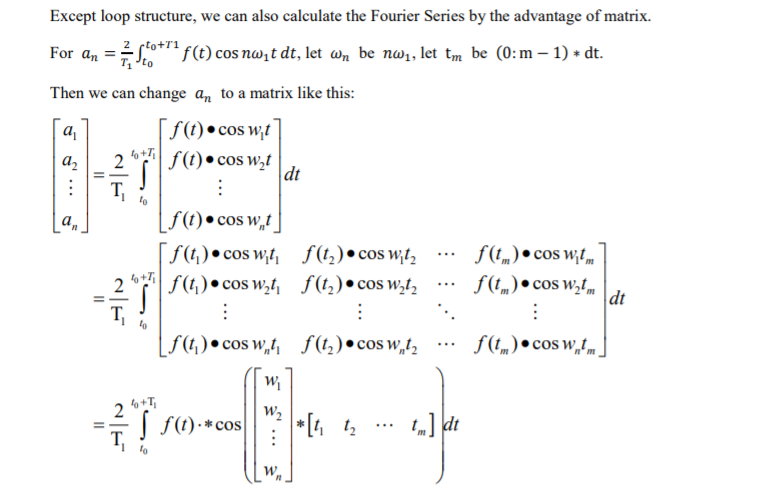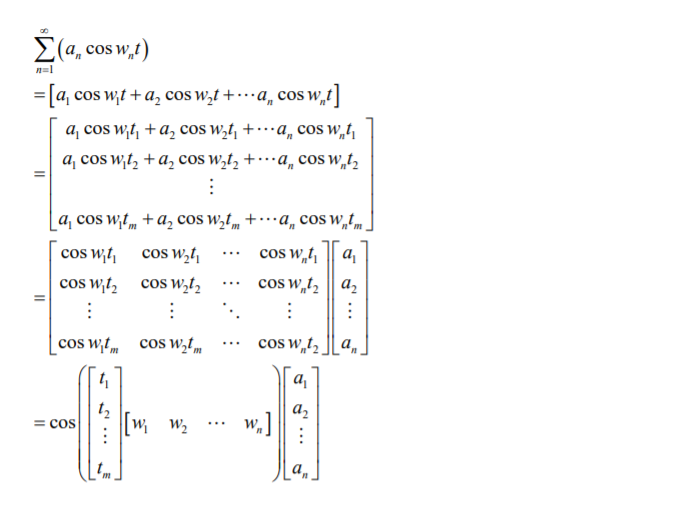% numeric method: matrix calculation
clear; clf;
T = 2; f = 1/T; w1 = 2*pi*f;
dt = 0.01;
t = -2:dt:2;
tao = -0.5:dt:0.5;
a0 = trapz(tao,tao.^0)/T;
N = input('N=');
n = 1:N;
fcos = 1.*cos(n'*w1*tao); an = trapz(tao,fcos,2)*2/T;
fsin = 1.*sin(n'*w1*tao); bn = trapz(tao,fsin,2)*2/T;
f = a0 + an'*cos(n'*w1*t) + bn'*sin(n'*w1*t);
plot(t,f); xlabel('t(s)');ylabel('ft'); grid on;
title(['Numeric Matrix with N=' num2str(N)]);


## Loop结构

% Symbolic method: loop function, int
clear; clf;
N = input('N=');
syms t1 a0 an bn n
T1 = 2; f = 1/T1; w1 = 2*pi*f;
range = [-0.5,0.5];
a0 = 1/T1*int(t1^0,t1,range);
f = a0;
for n=1:N
an = 2/T1*int(cos(n*w1*t1),t1,range);
bn = 2/T1*int(sin(n*w1*t1),t1,range);
f = f+an*cos(n*w1*t1)+bn*sin(n*w1*t1);
end
fplot(f); xlabel('t');ylabel('y(t)');
title(['Symbolic Loop with N=' num2str(N)]);
axis([-2,2,-0.2,1.2]); grid on;


## 吉布斯现象（Gibbs Phenomenon）

在进行高阶展开时。我们常常能遇到下面这些问题，在断点处的峰值不随N变大而减小，这就是所谓的吉布斯现象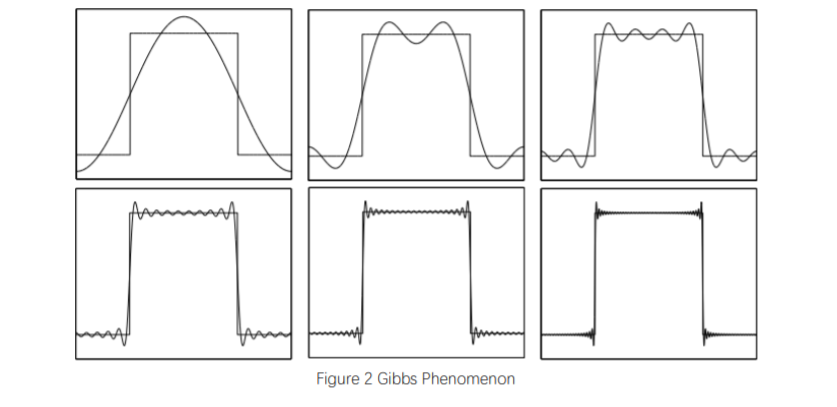## 另一种三角形式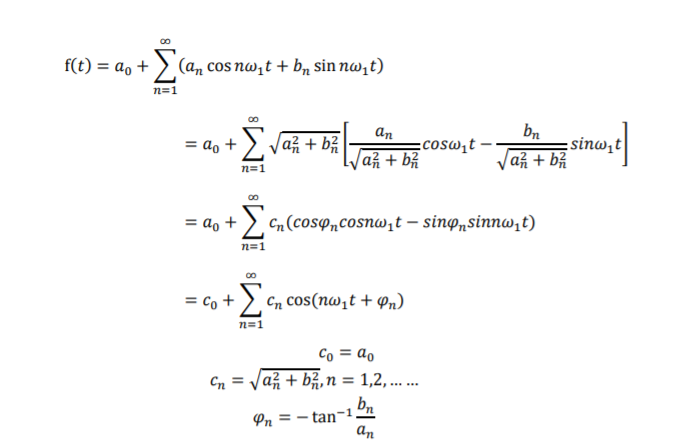## 指数形式（complex exponential form）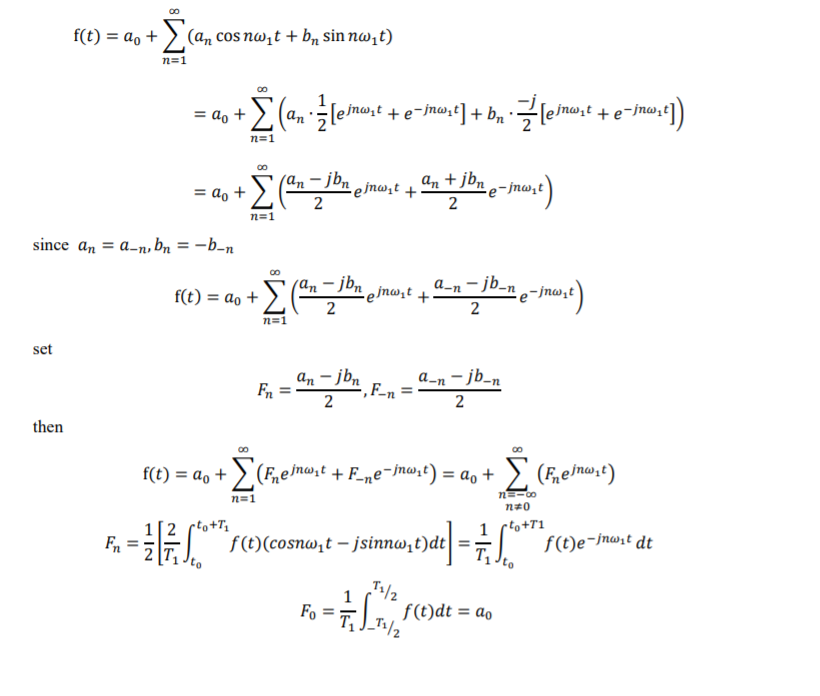## 三角与指数形式之间的关系## 样例（Sample）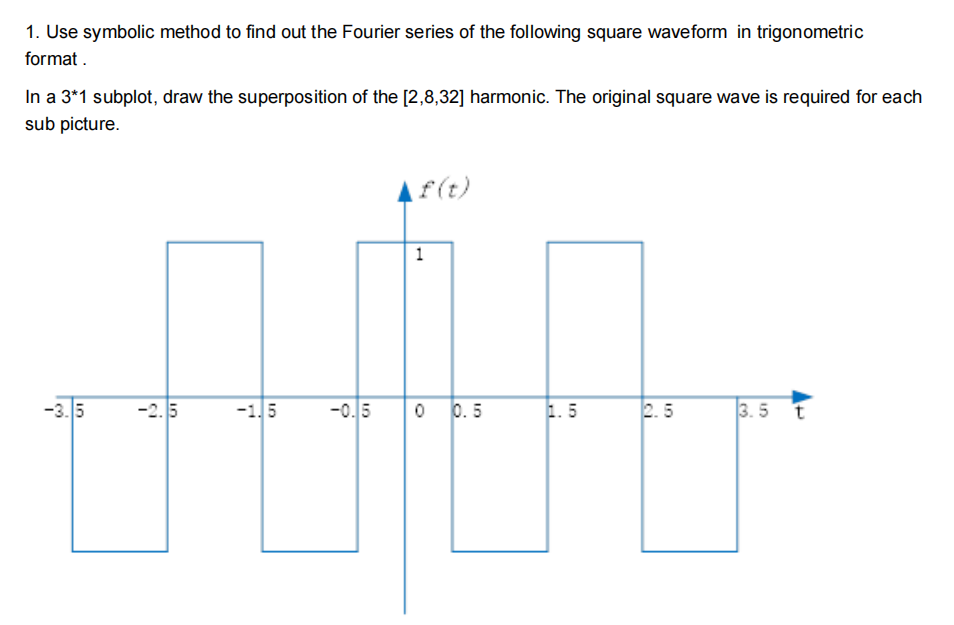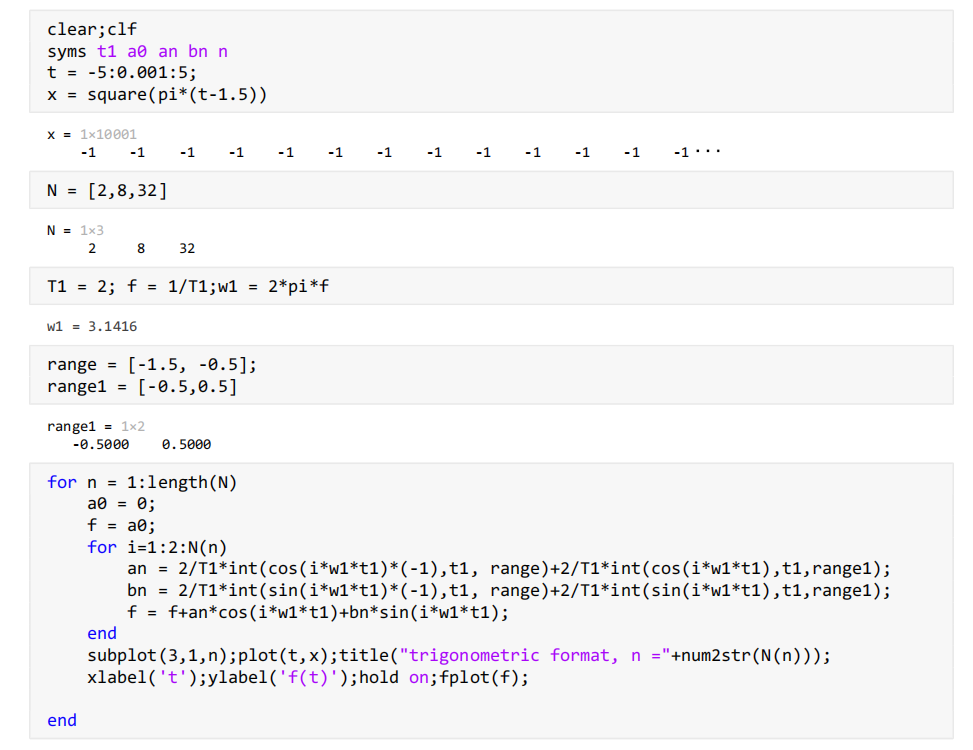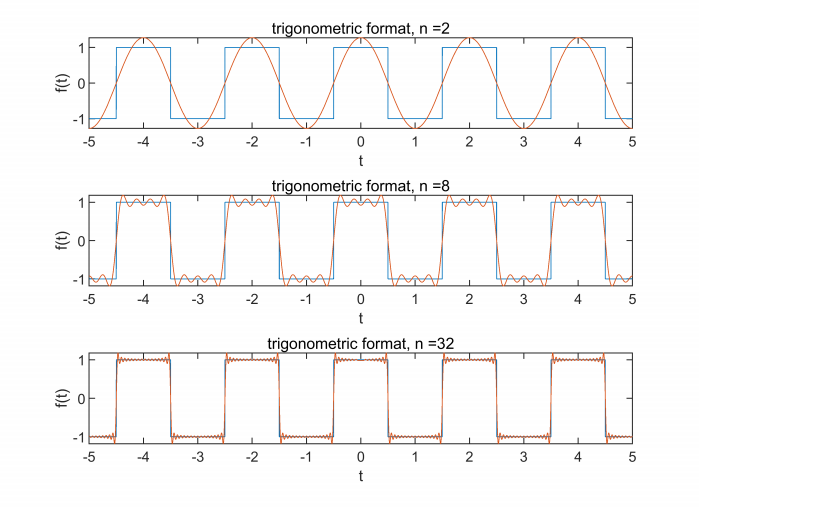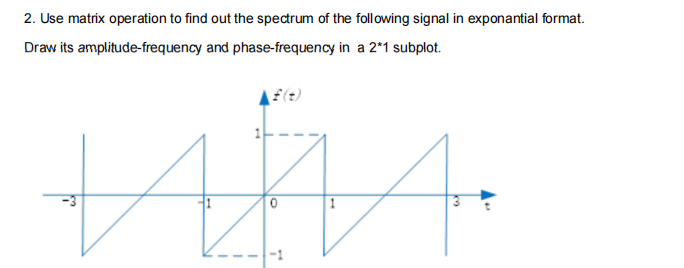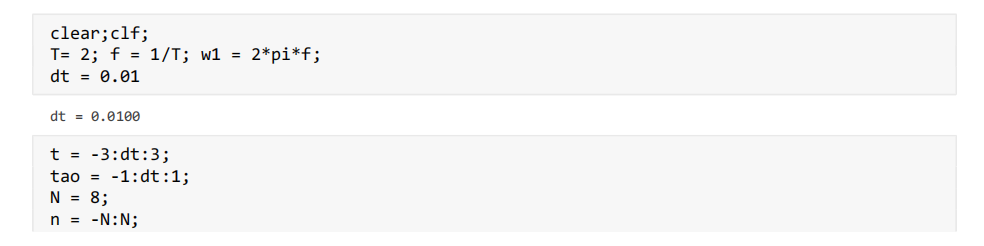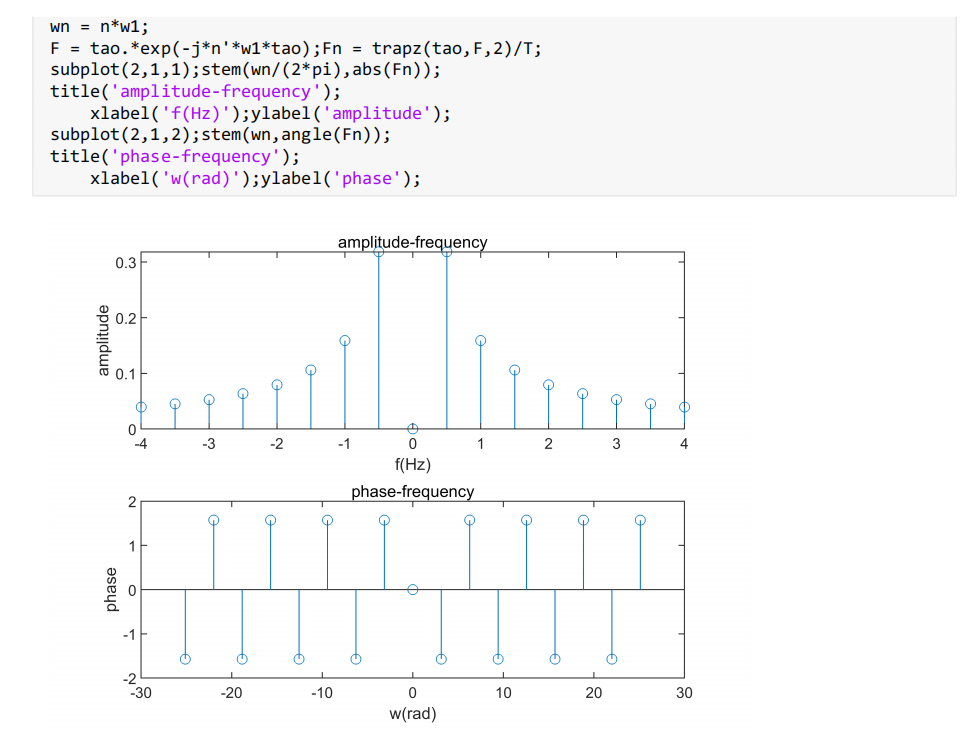展开全文matlab
• 目前为止并MATLAB并没有求解傅里叶级数的函数，但是可以利用int函数快速得到，详情见代码。 求解傅里叶级数及其图像逼近验证： syms x n; a0 = int(2,x,0,2)/2; a0 an = int(2*cos(n*pi*x/2),x,0,2)/2; an bn ...

目前为止并MATLAB并没有求解傅里叶级数的函数，但是可以利用int函数快速得到，详情见代码。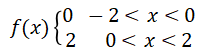求解傅里叶级数及其图像逼近验证：

syms x n;
a0 = int(2,x,0,2)/2;
a0
an = int(2*cos(n*pi*x/2),x,0,2)/2;
an
bn = int(2*cos(n*pi*x/2),x,0,2)/2;
bn
b = 40;
hold on
Sm = 1;
for n = 1:b
fn = 2*2*sin((2*n-1)*pi*x/2)/(2*n-1)/pi;
Sm =Sm +fn;
clf
subplot(2,1,1)
ezplot(fn,[-6,6])
subplot(2,1,2)
ezplot(Sm,[-6,6])
if n==6
pause
else
pause(5/n)
end
end

注：上述代码中有求解a0、an、bn公式。

输出：

a0 =

2

an =

(2*sin(pi*n))/(n*pi)

bn =

(2*sin(pi*n))/(n*pi)

图像：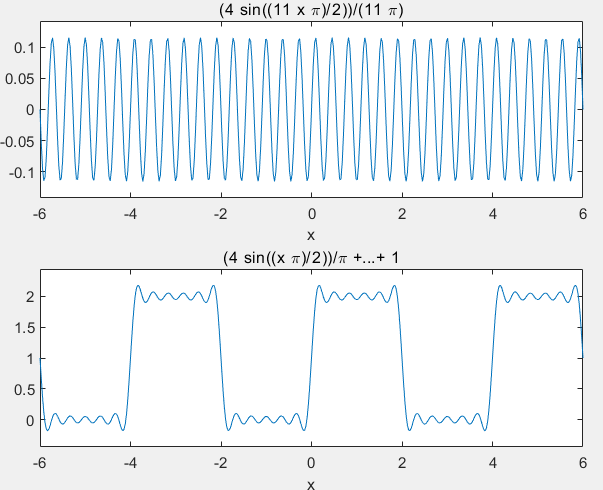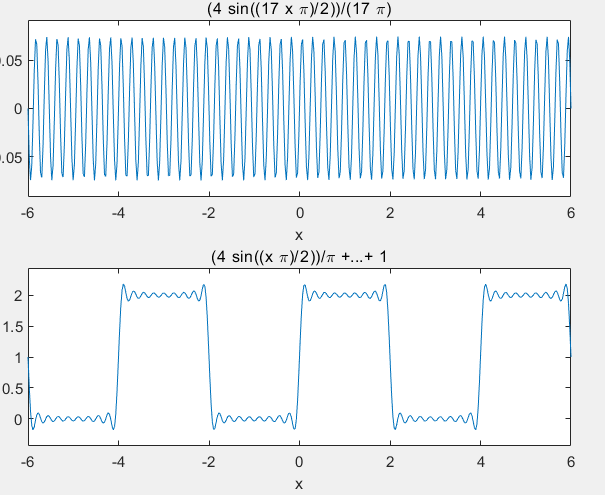可见随着b的增大，Sm图像逐渐逼近f(x)

展开全文matlab
• 此脚本允许您计算和可视化傅立叶级数的通用 f(x) 函数。 脚本很简单。 选择“a”值设置域扩展； 之后设置系列的顺序'n'，然后选择你的f（x）“f_x”。 该系列将以 3 种方式计算和表示：S_standard、S_armonic、S_...matlab
• Matlab函数逼近程序，包含下面所列多种算法的程序 。 Chebyshev 用切比雪夫多项式逼近已知函数 Legendre 用勒让德多项式逼近已知函数 Pade 用帕德形式的有理分式逼近已知...FZZ 用傅立叶级数逼近已知的连续周期函数
• 《数字信号处理实验 MATLAB版》 实验报告 山大实验11 离散傅里叶级数(DFS)(完美格式版，本人自己完成，所有语句正确，不排除极个别错误，特别适用于山大，勿用冰点等工具下载，否则下载之后的word格式会让很多部分...
• 程序 FFT2SURF 绘制双傅立叶级数表示用于几种不同的表面。 数字显示效果序列项的数量和 Lanczos 西格玛因子的使用平滑吉布斯振荡。 二次元的傅里叶级数周期为 px 和 py 的周期函数具有近似值形式： f(x,y) = sum(exp...matlab
• 实验三周期信号的傅里叶级数分析及MATLAB实现一、实验目的：1.利用MATLAB实现周期信号的分解与合成，并图示仿真结果；2.用MATLAB实现周期信号的频谱，画图观察和分析周期信号的频谱；3.通过MATLAB对周期信号频谱的...
• 2.用MATLAB实现周期信号的傅里叶级数分解与综合。 3.用MATLAB实现周期信号的单边频谱及双边频谱。 4.周期信号频谱与脉冲宽度和周期T之间的关系 二、实验内容 2、 三、实验总结 当脉冲信号周期不变，脉冲...matlab
• 1. 请绘出下面信号的幅频特性曲线2. 分别作出下面信号的幅频特性曲线和相频特性曲线，观察两个频谱的变化 3. 求下列频谱函数的傅里叶逆变换matlab
• 周期序列傅里叶级数Matlab实现 ** 周期序列离散傅里叶级数正变换: 周期序列离散傅里叶级数反变换: MATLAB实现： DFS式的矩阵形式： 周期序列的DFS定义，0≤n≤N-1，0≤k≤N-1 因此只需计算WN因子： 代码： ...
• 离散傅里叶级数：DFS 离散时间序列x(n)满足x(n)=x(n+rN)，称为离散周期序列，其中N为周期，x(n)为主值序列。 周期序列可用离散傅里叶级数表示成 n=0,1,…,N-1 其中，X ̃(k)是周期序列离散傅里叶级数第K次谐波分量...idft matlab
• 此应用程序允许用户定义分段函数，计算三角傅立叶级数展开的系数，并绘制近似值。matlab
• README 此程序为 2.0 版本，增加了实时 FFT 计算，目前整体功能已经调试完成，程序使用说明还没来得及整理，运行主函数 main.m 即可使用。 联系方式：知乎 程序截图MATLAB
• 程序将仅绘制傅立叶级数变换的任何分段函数......以't'作为变量......并且限制将是您喜欢的任何数字。 这是我们在信号处理中的最后一个项目之一……我希望这个程序也能帮助你加深对 Matlab 的理解……虽然我认为这...matlab
• 有关离散傅里叶级数（DFS）我之前也写过一些博文，例如：离散周期信号的傅里叶级数(DFS) 这里我再次给出标准公式。 分析式： 其中： 综合式： 这里我必须先声明，关于分析式和综合式前面那个系数1/N，...
• 周期信号的傅里叶级数 周期信号定义为随时间变换，取值呈周期变化的信号，即, k为整数，T称为信号的周期。一个正弦型信号源即为一个典型的周期信号。如果周期信号在一个周期内可积，则可以通过傅里叶级数展开该...matlab
•matlab
• Fourier con Matlab系列https://youtu.be/A4pPMjUCpu4matlab
• 函数 e^(-x) 在区间 (0,2pi) 上的傅立叶级数 (FS)。 这个程序类似于Matlab Demo of Square wave from Sine wave。 它绘制了 e^(-x) 和前 40 项 FS 之间的误差。 由于近似值（忽略 41 到 inf 项）而出现此错误matlab
• 一个非常简单的程序，可以使用傅立叶级数和周期函数。matlab
• matlab傅里叶级数展开程序，根据原函数表达式，求出傅里叶展开式
• 本人原创，感谢支持！本程序是最新的2.0版本。使用MATLAB，利用了FFT...利用MATLAB在绘图、计算方面的优势，将傅里叶的展开过程清晰地呈现出来，从而更好地帮助用户理解傅里叶级数，为电类专业未来的学习打下良好基础。
• 求矩形脉冲的傅里叶变换.. 过滤频谱并使用重新生成信号过滤后的频谱就完成了…… 最后通过证明时域和频域信号的能量含量相等，证明了瑞利定理。 您的意见是必需的.....matlab...